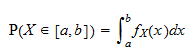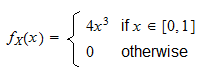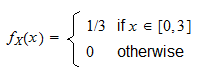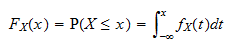StatLect

# Continuous random variable

A continuous random variable is a random variable having two main characteristics: 1) the set of values it can take is not countable; 2) its cumulative distribution function can be obtained by integrating a function called probability density function.## Synonyms

Continuous random variables are sometimes also called absolutely continuous.

## Definition

The following is a formal definition.

Definition A random variableis said to be continuous if and only if the probability that its realization will belong to an intervalcan be expressed as an integral:where the integrand functionis called the probability density function of.

Note that, as a consequence of this definition, the cumulative distribution function ofiswhich explains the introductory definition we have given.

## Examples

Let us give some examples (go to this lecture if you need to revise the basics of integration).

### Example 1

Letbe a continuous random variable that can take any value in the interval. Let its probability density function beThen, for example, the probability thattakes a value betweenandcan be computed as follows:### Example 2

Letbe a continuous random variable that can take any value in the intervalwith probability density functionThe probability that the realization ofwill belong to the intervalis## Properties

Continuous random variables have some interesting properties:

• their cumulative distribution functionis continuous because, as we have noted above, it can be expressed asand integrals are continuous with respect to their upper bound of integration.

• any single realizationhas zero probability of being observed becauseIf you are puzzled by this apparently paradoxical property, you are advised to read the lecture on zero-probability events.

• it follows from the definition given above that the support of a continuous random variable must be uncountable. In fact, by the previous property, if the support(the set of values the variable can take) was countable, then we would havewhich is a contradiction because the probability that a random variable takes at least one of all its possible values must be 1.

## Common continuous distributions

The next table contains some examples of continuous distributions that are frequently encountered in probability theory and statistics.

Name of the continuous distribution Support
Uniform All the real numbers in the interval [0,1]
Normal The whole set of real numbers
Chi-square The set of all non-negative real numbers

## More details

Continuous random variables are discussed in more detail in the lecture entitled Random variables.

You can also read a brief introduction to the probability density function, including some examples, in the glossary entry entitled Probability density function.